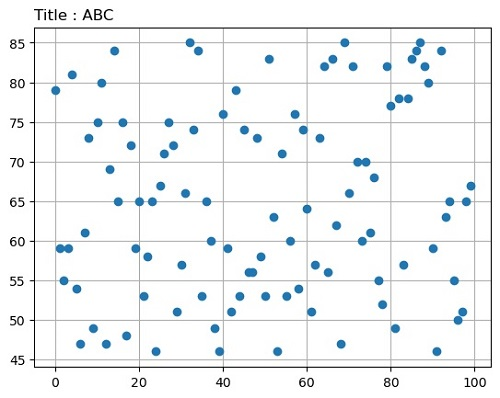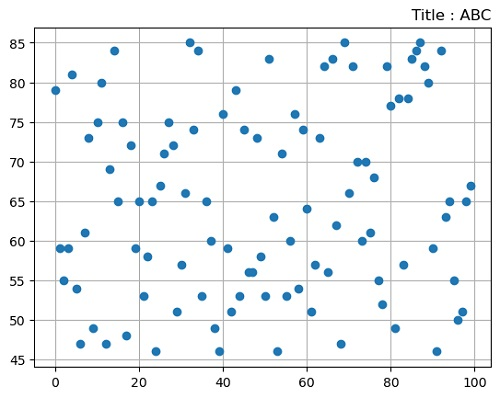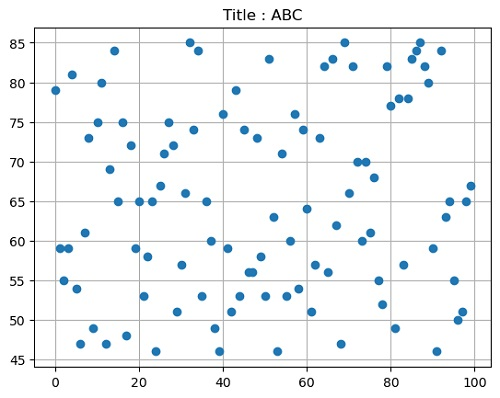# Python | Title Locations in Matplotlib

Here, we are going to learn about the title locations in matplotlib and its Python implementation.
Submitted by Anuj Singh, on August 04, 2020

In this tutorial, we are going to explore all three locations for the title of a Figure using matplotlib inbuilt command loc= ['left', 'right', 'center'].

Illustration:## Python code for title locations in matplotlib

```import numpy as np
import matplotlib.pyplot as plt

x = np.arange(100)
y = np.random.randint(45,86,100)

plt.figure()
plt.plot(x,y, 'o')
plt.title('Title : ABC', loc='left')
plt.grid()
plt.show()

plt.figure()
plt.plot(x,y, 'o')
plt.title('Title : ABC', loc='Right')
plt.grid()
plt.show()

plt.figure()
plt.plot(x,y, 'o')
plt.title('Title : ABC', loc='Center')
plt.grid()
plt.show()
```

Output:

```Output is as figure
```

Languages: » C » C++ » C++ STL » Java » Data Structure » C#.Net » Android » Kotlin » SQL
Web Technologies: » PHP » Python » JavaScript » CSS » Ajax » Node.js » Web programming/HTML
Solved programs: » C » C++ » DS » Java » C#
Aptitude que. & ans.: » C » C++ » Java » DBMS
Interview que. & ans.: » C » Embedded C » Java » SEO » HR
CS Subjects: » CS Basics » O.S. » Networks » DBMS » Embedded Systems » Cloud Computing
» Machine learning » CS Organizations » Linux » DOS
More: » Articles » Puzzles » News/Updates